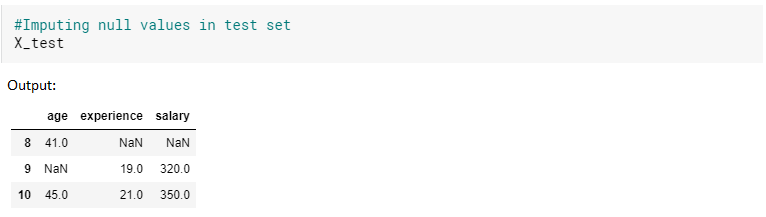top of pageSearch

# How to implement MICE algorithm using Iterative Imputer to handle missing values?

Any machine learning model is only as good as the data, so having a complete dataset with proper data is a must to develop a good model. Missing data often plagues real-world datasets, and hence there is tremendous value in imputing, or filling in, the missing values. Often the common methods like mean, median ,mode, frequent data and constant doesn’t provide correct data for the missing values.

To know more about MICE algorithm and it’s working check out my blog "MICE algorithm to Impute missing values in a dataset", in which I have explained in detail how MICE algorithm works with an example dataset.

In this blog, we will see how the MICE algorithm is implemented using the Scikit-learn Iterative Imputer. The Iterative Imputer was in the experimental stage until the scikit-learn 0.23.1 version, so we will be importing it from sklearn.experimental module as shown below.

Note:

If we try to directly import the Iterative Imputer from sklearn. impute, it will throw an error, as it is in experimental stage since I used scikit-learn 0.23.1 version.First, we need to import enable_iterative_imputer which is like a switch so that scikit-learn knows that we want to use the experimental version of Iterative Imputer.

I will use the same example that I used in my previous blog "MICE algorithm to Impute missing values in a dataset", so that it will be easy to understand as shown below:Let’s create the data frame for this data in the Kaggle notebook as shown below:Next, let’s remove the personal loan column as it is the target column and as we will not be imputing that column, we will not need it. We will work only with the feature columns as shown below:Now, let’s find out how the values are correlated to decide which algorithm to use to impute the null values.As we see here, the values we got are either 1 or very close to 1, so we can use linear regression to impute null values.Next, fit and transform the dataset with the imputer.In the above image, we can see that after we transform, the null values are imputed (circled numbers) and the dataset is shown in the form of an array.

This is how easy it is to impute null values using Iterative Imputer with very few lines of code.

## How to use Iterative Imputer in the case of training and testing sets?

To demonstrate the working of Iterative Imputer in the case of training and testing sets, we will use the same dataset with more records as shown below:Next, let’s remove the personal loan column as it is the target column and as we will not be imputing that column, we will not need it.Next, let’s split the dataset in to train and test datasets using train_test_split function.Now, let's repeat the same steps we did earlier for the whole dataset, using linear regression as the imputer model and we will fit and transform the training dataset with that imputer as shown.As we can see, the null values in the training dataset are imputed (circled numbers).

Finally, let’s do the same on the test set to impute the null values. The test set is as follows:For the test set, we should just use the same imputer that we used for the train set and call the transform function on the test set. We should not again define a new imputer for the test set.As shown in the above image, the null values in the test set are now imputed(circled numbers) and thus we have imputed all the null values in both training and testing sets easily without much difficulty.

In a nutshell, this is how the Iterative imputer works to impute the null values in a dataset and we can see how effortlessly we could impute the missing values using the same.

Hope this would be useful to everyone who is working with datasets that has missing values and trying to fill appropriate data for those, so that the model that uses that dataset could predict accurately.

Happy Imputing!